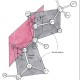Introduction

Let$X_1$,$X_2$, …,$X_m$ be independent random variables with an exponential distribution. We have already found the expression of the distribution of the random variable Y =$X_1$ +$X_2$ + …+$X_m$ when$X_1>0$,$X_2>0$, …,$X_m>0$ have pairwise distinct parameters (here). The reader will easily recognize that the formula we found in that case has no meaning when the parameters are all equal to λ. I will derive here the law of Y in this circumstance. This can be done with a demonstration by induction, with no particular effort, but I will follow a longer proof. Why? Perhaps because I like pain? Yes, this might be true; but the main reason is that, to me,  this longer demonstration is quite interesting and it gives us the chance to introduce the following proposition. In this blog post, we will use some of the results from the previous one on the same topic and we will follow the same enumeration for propositions.

PROPOSITION 8 (sum of m independent random variables). Let$X_1$,$X_2$, …,$X_m$ be independent random variables. The law of Y =$X_1$ +$X_2$ + …+$X_m$ is given by:

Proof. We know that the thesis is true for m=2 (PROP. 1). Consider now that:

But we know that$X_1$,$X_2$, …,$X_m$ are independent. So we have:

We now operate the substitution

And we obtain:

But this has to be true for any possible interval [a, b], which means that:

This is one of the equations in the thesis. The other ones can be derived in the same way ♦

Guessing the solution

I will solve the problem for m = 2, 3, 4 in order to have an idea of what the general formula might look like.

PROPOSITION 9 (m=2). Let$X_1$,$X_2$ be independent exponential random variables with the same parameter λ. The law of$Y_1$ =$X_1$ +$X_2$ is given by:

for y > 0. It is zero otherwise.

Proof. We just have to substitute$f_{X_1}$,$f_{X_2}$ in Prop. 1. We obtain:

And we find the thesis by solving the integral ♦

PROPOSITION 10 (m=3). Let$X_1$,$X_2$,$X_3$ be independent exponential random variables with the same parameter λ. The law of Y =$X_1$ +$X_2$ +$X_3$ is given by:

for y>0. It is zero otherwise.

Proof. If we define$Y_1$ =$X_1$ +$X_2$ and$Y_2$=$X_3$, then we can say – thanks to Prop. 2 – that$Y_1$ and$Y_2$ are independent. This means that – according to Prop. 1 – we have

And the proof is concluded ♦

PROPOSITION 11 (m=4). Let$X_1$,$X_2$,$X_3$,$X_4$ be independent exponential random variables with the same parameter λ. The law of Y =$X_1$ +$X_2$ +$X_3$ +$X_4$ is given by:

Proof. We can demonstrate the thesis in the same way we did in Prop. 10. We can draw the same conclusion by directly applying Prop. 8:Figure 1. This tetrahedron is defined by the conditions and for i = 2, 3, 4. It is the domain of integration for the integral in the proof of proposition 11.

The domain of integration is the tetrahedron in Figure 1. The generic point P within the tetrahedron has to belong to the segment AC that, in turns, belongs to the triangle shaded grey. This means that the domain of integration can be written [0, y]×[0, y$x_2$]×[0, y$x_2$$x_3$]. Thus, we calculate:

and the proof is concluded ♦

Proof

The reader has now likely guessed what the density of Y looks like when m is the generic integer number. But before we can rigorously demonstrate that formula, we need to calculate an integral.

PROPOSITION 12 (lemma). It is true that:

Proof. We have already found that the thesis is true when m = 4. Let us now assume that it is true for m – 1; if we write the thesis for the m – 2 variables$x_3$,$x_4$, … ,$x_m$ we have:

Now we put η = y$x_2$:

By integrating both members in [0, y] we obtain:

and the proof is concluded ♦

PROPOSITION 13. Let$X_1$,$X_2$, …,$X_m$ be independent random variables. The law of Y =$X_1$ +$X_2$ + …+$X_m$ is given by:

Proof. By directly applying Prop. 8 we have:

But:

Thus, we can write:

The domain of integration can be written in a more proper way (as we did in Prop. 11) as follows:

But this is the integral calculated in Prop. 12, and the proof is concluded ♦

A numerical application

The reader might have recognized that the density of Y in Prop. 13 is, in fact, a Gamma distribution: it is the distribution Γ(m, λ), also known as Erlang’s distribution. There might even be a reader who perhaps remembers that I have discussed that distribution in a post of mine (here) and that I have coded a program that plots both the density and the distribution of such a law. In Figure 2 we see the density of Y (left) and the distribution function (right) for λ = 1.5 and for m that goes from 1 to 3 (note that m is indicated with α in that picture).Figure 2. Density (left) and distribution function (right) of Y for λ = 1.5 and for m that goes from 1 to 3 (note that m is indicated with α in this picture).

## 4 thoughts on “Sum of independent exponential random variables with the same parameter”

1.Alex says:

Molto interessante.
Nella dimostrazione della Prop 11 come si passa da integrazione da su tutto R3 a integrazione nel tetraedro?
Credo si debba imporre$y-x_2-x_3-x_4>0$ e$x_i>0$

Liked by 1 person

1.Paolo Maccallini says:

Sì, è corretto. La prima condizione che hai indicato individua il semispazio che include l’origine, dei due in cui lo spazio è diviso dal piano$\pi$. Insieme alle altre tre condizioni menzionate ($x_i>0$ per i = 2, 3, 4), resta individuato il tetraedro. Qui, come in altri articoli, alcuni passaggi e spiegazioni sono stati saltati. Grazie per il commento!

Like

2.Paolo Maccallini says:

Questa dimostrazione, insieme a molte altre relative alle densità discrete e continue, si trova in un volume che sto scrivendo. Nel link seguente è possibile consultare e scaricare una versione già molto elaborata del testo: